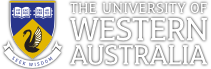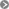CITS2002 Systems ProgrammingCITS2002CITS2002 schedule

### Introducing functions

C is a procedural programming language, meaning that its primary synchronous control flow mechanism is the procedure call.

C names its procedures functions (in contrast, Java has a different mechanism - methods).

• In Mathematics, we apply a function, such as the trigonometric function cos, to one or more values.
The function performs an evaluation, and returns a result.

• In many programming languages, including C, we call or invoke a function.
We evaluate zero or more expressions, the result of each expression is copied to a memory location where the function can receive them as arguments, the function's statements are executed (often involving the arguments), and a result is returned (unless the function is stuck in an infinite-loop or exits the process!)

We've already seen the example of main() - the function that all C programs must have, which we might write in different ways:
 ``` #include #include int main(int argcount, char *argvalue[]) { // check the number of arguments if(argcount != 2) { .... exit(EXIT_FAILURE); } else { .... exit(EXIT_SUCCESS); } return 0; } ```

 ``` #include #include int main(int argcount, char *argvalue[]) { int result; // check the number of arguments if(argcount != 2) { .... result = EXIT_FAILURE; } else { .... result = EXIT_SUCCESS; } return result; } ```

The operating system calls main(), passing to it some (command-line) arguments, main() executes some statements, and returns to the operating system a result - usually EXIT_SUCCESS or EXIT_FAILURE.

CITS2002 Systems Programming, Lecture 5, p1, 12th August 2019.

### Why do we require functions?

The need for, and use of, main() should be clear. However, there's 4 other primary motivations for using functions:

1. Functions allow us to group together statements that have a strongly related purpose - statements, in combination, performing a single task.

We prefer to keep such statements together, providing them with a name (as for variables), so that we may refer to the statements, and call them, collectively.

This provides both convenience and readability.

2. We often have sequences of statements that appear several times throughout larger programs.

The repeated sequences may be identical, or very similar (differing only in a very few statements). We group together these similar statement sequences, into a named function, so that we may call the function more than once and have it perform similarly for each call.

• Historically, we'd identify and group similar statements into functions to minimize the total memory required to hold the (repeated) statements.
• Today, we use functions not just to save memory, but to enhance the robustness and readability of our code (both good Software Engineering techniques).

3. From the Systems Programming perspective, the operating system kernel employs functions as well-defined entry points from user-written code into the kernel.

Such functions are named system calls.

4. Functions provide a convenient mechanism to package and distribute code. We can distribute code that may be called by other people's code, without providing them with a complete program.

We frequently use libraries for this purpose.

CITS2002 Systems Programming, Lecture 5, p2, 12th August 2019.

### Where do we find functions?

1. We'll initially write our own functions, in the same file as main(), to simplify our code and to make it easier to read.

2. Soon, we'll write our own functions in other, multiple files, and call them from our main file.

3. Collections of related functions are termed libraries of functions.

The most prominent example, that we've already seen, is C's standard library - a collection of frequently required functions that must be provided by a standards' conforming C compiler.

In our programming, so far, we've already called library functions such as:

printf(),  atoi(),  and  exit().

4. Similarly, there are many task-specific 3rd-party libraries. They are not required to come with your C compiler, but may be downloaded or purchased - from Lecture 1 -

Function domain 3rd-party libraries
operating system services
(files, directories, processes, inter-process communication)
OS-specific libraries, e.g. glibc, Win32, Carbon
web-based programming libcgi, libxml, libcurl
data structures and algorithms the generic data structures library (GDSL)
GUI and graphics development OpenGL, GTK, Qt, UIKit, Win32, Tcl/Tk
image processing (GIFs, JPGs, etc) gd
networking Berkeley sockets, AT&T's TLI
security, cryptography openssl, libmp
scientific computing NAG, Blas3, GNU scientific library (gsl)
concurrency, parallel and GPU programming pthreads, OpenMPI, openLinda, CUDA, OpenCL

CITS2002 Systems Programming, Lecture 5, p3, 12th August 2019.

### The role of function main()

In general, small programs, even if just written in a single file, will have several functions.

We will no longer place all of our statements in the main() function.

main() should be constrained to:

• receive and check the program's command-line arguments,

• call functions from main(), typically passing information requested and provided by the command-line arguments, and

• finally call exit(EXIT_SUCCESS) if all went well.

And, in a forthcoming lecture, the following will make more sense:

• All error messages printed to the stderr stream.

• All 'normal' output printed to the stdout stream (if not to a requested file).

CITS2002 Systems Programming, Lecture 5, p4, 12th August 2019.

### The datatype of a function

There are two distinct categories of functions in C:

1. functions whose role is to just perform a task, and to then return control to the statement (pedantically, the expression) that called it.

Such functions often have side-effects, such as performing some output, or modifying a global variable so that other statements may access that modified value.

These functions don't return a specific value to the caller, are termed void functions, and we casually say that they "return void".

2. functions whose role is to calculate a value, and to return that value for use in the expressions that called them. The single value returned will have a type, such as int, char, bool, or float.

These functions may also have side-effects.

 ``` #include #include void output(char ch, int n) { for(int i=1 ; i<=n ; i=i+1) { printf("%c", ch); } } int main(int argcount, char *argvalue[]) { output(' ', 19); output('*', 1); output('\n', 1); return 0; } ```

 ``` #include #include #include float square(float x) { return x * x; } int main(int argcount, char *argvalue[]) { if(argcount > 2) { float a, b, sum; a = atof(argvalue); b = atof(argvalue); sum = square(a) + square(b); printf("hypotenuse = %f\n", sqrt(sum) ); } return 0; } ```

Note that this 2nd example uses the sqrt() function from the standard maths library.
We should thus compile it with:  cc [EXTRAOPTIONS] -o program program.c -lm

CITS2002 Systems Programming, Lecture 5, p5, 12th August 2019.

### Passing parameters to functions

The examples we've already seen show how parameters are passed to functions:

• a sequence of expressions are separated by commas, as in:

a = average3( 12 * 45, 238, x - 981 );

• each of these expressions has a datatype. In the above example, each of the expressions in an int.
• when the function is called, the expressions are evaluated, and the value of each expression is assigned to the parameters of the function:

 ``` float average3( int x, int y, int z ) { return (x + y + z) / 3.0; } ```

• during the execution of the function, the parameters are local variables of the function.

• They have been initialized with the calling values (x = 12 * 45 ...), and the variables exist while the function is executing.
They "disappear" when the function returns.

• Quite often, functions require no parameters to execute correctly. We declare such functions with:

 ``` void backup_files( void ) { ..... } ```

and we just call the functions without any parameters: backup_files();

CITS2002 Systems Programming, Lecture 5, p6, 12th August 2019.

### Very common mistakes with parameter passing

Some common misunderstandings about how parameters work often result in incorrect code
(even a number of textbooks make these mistakes!):

• The order of evaluation of parameters is not defined in C. For example, in the code:

 ``` int square( int a ) { printf("calculating the square of %i\n", a); return a * a; } void sum( int x, int y ) { printf("sum = %i\n", x + y ); } .... .... sum( square(3), square(4) ); ```

are we hoping the output to be:

```    calculating the square of 3     // the output on PowerPC Macs
calculating the square of 4
sum = 25

or

calculating the square of 4     // the output on Intel Macs
calculating the square of 3
sum = 25
```

Do not assume that function parameters are evaluated left-to-right. The compiler will probably choose the order of evaluation which produces the most efficient code, and this will vary on different processor architectures.

(A common mistake is to place auto-incrementing of variables in parameters.)

CITS2002 Systems Programming, Lecture 5, p7, 12th August 2019.

### Very common mistakes with parameter passing, continued

• Another common mistake is to assume that function arguments and parameters must have the same names to work correctly.

Some novice programmers think that the matching of names is how the arguments are evaluated, and how arguments are bound to parameters.
For example, consider the code:

 ``` int sum3( int a, int b, int c ) { return a + b + c; } .... int a, b, c; a = 1; b = 4; c = 9; printf("%i\n", sum3(c, a, b) ); ```

Here, the arguments are not "shuffled" until the names match.

It is not the case that arguments must have the same names as the parameters they are bound to.
Similarly, the names of variables passed as arguments are not used to "match" arguments to parameters.

If you ever get confused by this, remember that arithmetic expressions, such as 2*3 + 1, do not have names, and yet they are still valid arguments to functions.

CITS2002 Systems Programming, Lecture 5, p8, 12th August 2019.

### Very common mistakes with parameter passing, continued

• While not an example of an error, you will sometimes see code such as:

```        return x;
```

and sometimes:

```        return(x);
```

While both are correct, the parentheses in the 2nd example are unnecessary.

return is not a function call, it is a statement, and so does not need parentheses around the returned value.

However - at any point when writing code, use extra parentheses if they enhance the readability of your code.

CITS2002 Systems Programming, Lecture 5, p9, 12th August 2019.

### Functions receiving a variable number of arguments

To conclude our introduction to functions and parameter passing,
we consider functions such as printf() which may receive a variable number of arguments!

We've carefully introduced the concepts that functions receive strongly typed parameters, that a fixed number of function arguments in the call are bound to the parameters, and that parameters are then considered as local variables.

But, consider the perfectly legal code:

 ```#include int i = 238; float x = 1.6; printf("i is %i, x is %f\n", i, x); .... printf("this function call only has a single argument\n"); .... printf("x is %f, i is %i, and x is still %f\n", x, i, x); ```

In these cases, the first argument is always a string, but the number and datatype of the provided arguments keeps changing.

printf() is one of a small set of standard functions that permits this apparent inconsistency. It should be clear that the format specifiers of the first argument direct the expected type and number of the following arguments.

Fortunately, within the ISO-C99 specification, our cc compiler is permitted to check our format strings, and warn us (at compile time) if the specifiers and arguments don't "match".

 ``` prompt> cc -o try try.c try.c:9:20: warning: format specifies type 'int' but the argument has type 'char *' [-Wformat] printf("%i\n", "hello"); ~~ ^~~~~~~ %s 1 warning generated. ```

CITS2002 Systems Programming, Lecture 5, p10, 12th August 2019.